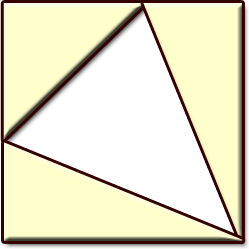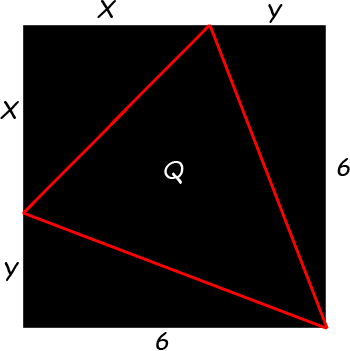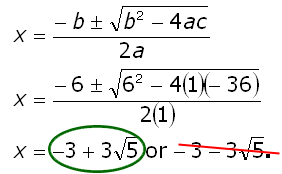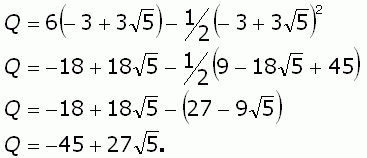SEARCH HOMEMath Central Quandaries & QueriesQuestion from Kristina, a student: A square with side lengths of 6 cm is divided into 3 right triangles and a larger isosceles triangle. If the three right triangles have equal area, find the exact area of the isosceles triangle.Kristina,

Try this shape.Stephen La Rocque.

If you need more help, scroll down.

Determine the three variable relationships:This diagram labels the lengths that we need to consider as well as the area Q of the large isosceles triangle. We have three variables, so we need three distinct equations to solve the problem. They are:

(1) Side length: x + y = 6

(2) Equal areas: ½x2 = 3y

(3) Total area: ½x2 + 3y + 3y + Q = 62.

Scroll down if you still need more hints.

Get rid of y:
Combine the first two equations using the substitution method:

 ½x2 = 3y x + y = 6 x2 - 6y = 0 y = 6 - x x2 - 6(6 - x) = 0 x2 + 6x - 36 = 0

Now use the Quadratic Formula to solve for x. Since x is a length, we can ignore the negative solution and choose just the positive solution.

Scroll down for more of the solution.Next, we can combine equations (1) and (3) to solve for Q, the area we have been asked to find:

 ½x2 + 3y + 3y + Q = 62 x+y=6 Q = 36 - ½x2 - 6y y=6-x Q = 36 - ½x2 - 6(6-x) Q = 6x - ½x2

And finally, substitute the value for x that we calculated earlier into this expression.

Final calculation:Solved. You can check by calculating y and the area of each triangle and seeing that they add to 36 square centimeters.

Stephen.Math Central is supported by the University of Regina and The Pacific Institute for the Mathematical Sciences.